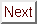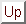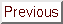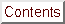Next: Estimation of the Up: No Title Previous: Estimation of the

# Effect of Tilt on Angular Dependence of the Critical Field

Effect of Tilt

In this section, a detailed derivation of Eqn.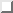is presented. In order to understand the geometry of the sample tilt, the reader is referred to Figure.

The underlying assumption of the derivation presented here is that the material in question has uniaxial symmetry. In the current context, uniaxial symmetry means that the material has a plane in which Hc2 is constant. The angle between the perpendicular to this plane and the magnetic field is called theta.

For graphite-based superconductors, the planes of constant Hc2 are those parallel to the graphite layers, and the perpendicular axis which defines the angle theta is called the c-axis. (The angle theta is defined in Figure.) In-plane isotropy has not actually been experimentally confirmed in GIC superconductors because of the difficulty of preparing single-crystal samples. However, any in-plane anisotropy is expected to be small because the in-plane band structure should be relativelty free-electron-like. In addition, the critical field experiments discussed in this thesis were performed on HOPG-based samples. Since HOPG is composed of randomly oriented crystallites in-plane, but has a well-defined c-axis direction, GIC's prepared from HOPG should meet the definition of a uniaxial superconductor even if in-plane anisotropy is present in single crystals.

The derivation of Eqn.follows the simple derivation of Eqn.that was published by Morris, Coleman, and Bhandari. Let's first review their derivation. These authors started with the idea that a the coherence length of a uniaxial superconductor in any direction is equivalent to the length of a vector joining the center of a biaxial ellipsoid with its edge. The ellipsoid has a circular cross-section of radius xia, which corresponds to the circular cross-section of a flux quantum formed when vecH || ^c. The elliptical cross-section of the ellipsoid corresponds to the case where vecH _|_ ^c, where the flux quantum has radii xia and epsilon xia. Here epsilon is the AGL model anisotropy parameter introduced in Ref. . The biaxial ellipsoid is shown in Figurea), and its two cross-sections in Figurea).

For any orientation of the applied field, one of the coherence lengths in the plane perpendicular to the field will be xia, the in-plane coherence length. The other coherence length in this plane will vary continuously with theta from xia to epsilon xia. Thususing Eqn.. Figurea) and some simple geometrical manipulations show that xi(theta) = xia sqrtcos2 theta + epsilon2 sin2 theta . Plugging the xi (theta) expression into Eqn.above gives Eqn.:as expected. This is the result obained by Morris, Coleman, and Bhandari.Figure: Coherence lengths of a uniaxial superconductor. As pointed out by Morris et al., the coherence length of a uniaxial superconductor is the length of a vector from the center of a biaxial ellipsoid to its edge. a) The case of an aligned sample, which is described by Eqn.. (See Figurea).) The ellipsoid has two radii of length xia and one of length epsilon xia. b) The case where the sample is tilted by an angle ø, which is described by Eqn.. Now the ellipsoid is triaxial, with one of the coherence lengths of size xia from a) being replaced by one of size xia sqrtcos2 ø + epsilon2 sin2 ø.Figure: Cross-section of the flux quantum in a uniaxial superconductor. In all cases, the magnetic field is directed out of the paper. a) The aligned case. For vecH || ^c, the cross-section of the flux quantum along the field direction is circular. b) The tilted case. Now that the ellipsoid that determines xi(theta) is triaxial, the cross-section of the flux quantum is non-circular for all field orientations.

Now let's turn to the case where the sample is tilted so that its c-axis is rotated by an angle ø away from the horizontal plane, which is the plane in which the applied field is rotated. From examination of Eqn., it is obvious that the measured magnitude of Hc2, || ^c will be Hc2, || ^c eff == Hc2,|| ^c/ sqrtcos2 ø + epsilon 2 sin2 ø. Note that this gives Hc2, || ^ceff = Hc2, || ^c when ø = 0°. If Hc2(theta) still is given by Eqn., then it follows that xi(0°) = xia sqrtcos2 ø + epsilon2 sin2 ø.

Now that xi(theta) has been found for theta = 0°, the full form of the function can be determined by noting that xi(90°) still is epsilon xia. The reason is that the tilt of the c-axis from the horizontal corresponds to a rotation about an in-plane direction (an ^a direction), so the in-plane Hc2 is not affected by the tilt. This statement is no longer true if Hc2 is not constant in the layer plane (non-uniaxial superconductor), although everything said up to this point holds for that case. Using standard formulae for an ellipse gives for general thetawhere epsiloneff = epsilon / sqrtepsilon2 sin2 ø + cos 2 ø. Plugging this formula for xi(theta) into Eqn.now gives Eqn.:as desired.Next: Estimation of the Up: No Title Previous: Estimation of the

alchaiken@gmail.com (Alison Chaiken)
Wed Oct 11 22:59:57 PDT 1995